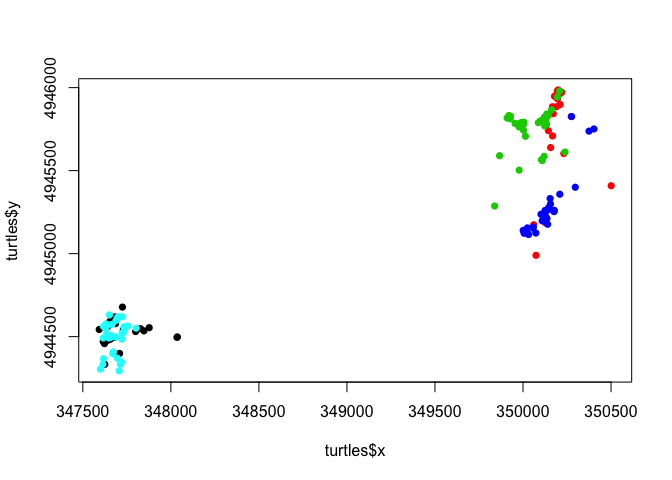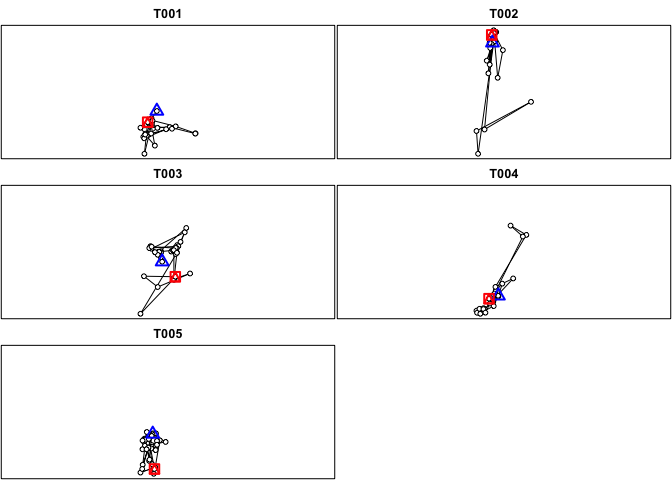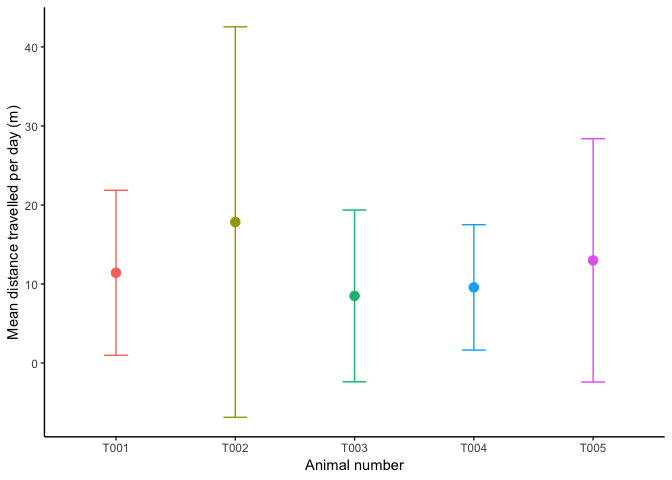What is a trajectory and why is it useful? A trajectory is the path connecting the relocations of a tracked animal. Aspects of the trajectory, such as the distance between each relocation and the direction (angle) of each segment, are very useful for modeling animal movement and testing predictions of hypotheses.

In the last post, I formatted telemetry data for analyses. In this post, I will show one way of creating and analyzing animal paths from telemetry data, using some simulated data on turtles.

The data are in a csv file (`tracking_sample.csv`).

``````# Read the csv file
stringsAsFactors = FALSE)
# The file should be in your working directory.

# Examine the structure of the data. Note presence of some NA's
str(turtles)``````
``````## 'data.frame':    170 obs. of  6 variables:
##  \$ id  : chr  "T001" "T001" "T001" "T001" ...
##  \$ date: chr  "2013-07-07" "2013-07-12" "2013-07-21" "2013-07-28" ...
##  \$ time: chr  "9:24:00 AM" "8:57:00 AM" "9:53:00 AM" "11:30:00 AM" ...
##  \$ x   : int  347725 347670 347682 347662 347877 348037 348035 347828 347593 347635 ...
##  \$ y   : int  4944678 4944599 4944619 4944609 4944554 4944498 4944496 4944548 4944543 4944489 ...
##  \$ zone: int  18 18 18 18 18 18 18 18 18 18 ...``````

We can check everything is working by visualizing our data.

``````plot(turtles\$y~turtles\$x,
col = as.factor(turtles\$id),
pch = 16)``````To create trajectories, we don’t need to create a SpatialPointsDataFrame and can work directly with the dataframe.

Let’s examine how we can quantify the path, or trajectory of the tracked animals. The function `as.ltraj` in the `adehabitatLT` package makes this easy.

To start, we need to give `as.ltraj` three arguments:

• xy: the coordinates
• date: a POSIXct object with date and (if present) time
• id: a variable to group points based on individual

Note: If you want distances calculated in metres, you should have your coordinates in UTM. For converting between coordinate types, you can use the `spTransform` function in the `sp` package.

``````# Load library
# You will need to install the package the first time with:
``````# We need to make sure that date is correctly formatted, and that there is an ID column
turtles.ltraj <- as.ltraj(xy = turtles[,c("x", "y")],
date =  as.POSIXct(paste(turtles\$date, turtles\$time, sep = " ")),
id = turtles\$id)``````

In other data sets, it is common to receive an error saying “non unique dates for a given burst”

Two common issues in real telemetry data cause this error:

1. Some combinations of date & time are repeated for an animal (because of a mistake in data entry)
2. Time is incorrectly formatted and `as.POSIXct` is dropping the time section.

If you’re receiving that error, look into those issues first.

``plot(turtles.ltraj) # Plots each animal's points with a path connecting them````turtles.ltraj  # data.ltraj is a list``
``````##
## *********** List of class ltraj ***********
##
## Type of the traject: Type II (time recorded)
## * Time zone unspecified: dates printed in user time zone *
## Irregular traject. Variable time lag between two locs
##
## Characteristics of the bursts:
##     id burst nb.reloc NAs          date.begin            date.end
## 1 T001  T001       29   0 2013-07-07 09:24:00 2015-05-09 07:57:00
## 2 T002  T002       33   0 2013-07-06 11:13:00 2014-10-26 11:27:00
## 3 T003  T003       40   0 2013-07-07 10:33:00 2015-10-08 07:21:00
## 4 T004  T004       28   1 2013-07-13 09:00:00 2014-10-26 08:57:00
## 5 T005  T005       40   1 2013-07-05 10:59:00 2015-09-07 08:54:00
##
##
##  infolocs provided. The following variables are available:
##  "pkey"``````
``````# Each element of the list is a dataframe for one individual
head(turtles.ltraj[])  # The first six locations of the first animal``````
``````##        x       y                date  dx  dy       dist     dt    R2n
## 1 347725 4944678 2013-07-07 09:24:00 -55 -79  96.260064 430380      0
## 2 347670 4944599 2013-07-12 08:57:00  12  20  23.323808 780960   9266
## 3 347682 4944619 2013-07-21 09:53:00 -20 -10  22.360680 610620   5330
## 4 347662 4944609 2013-07-28 11:30:00 215 -55 221.923410 510840   8730
## 5 347877 4944554 2013-08-03 09:24:00 160 -56 169.516961 515400  38480
## 6 348037 4944498 2013-08-09 08:34:00  -2  -2   2.828427 785160 129744
##    abs.angle   rel.angle
## 1 -2.1789691          NA
## 2  1.0303768 -3.07383938
## 3 -2.6779450  2.57486344
## 4 -0.2504431  2.42750195
## 5 -0.3366748 -0.08623173
## 6 -2.3561945 -2.01951967``````

The trajectory for each animal measures:

• distance,
• dt (the difference in seconds between relocations),
• R2n (the squared distance between the first relocation of the trajectory and the current relocation), and
• two angle measurements (absolute and relative).

For a complete description of these parameters, check-out the package tutorial (type `vignette("adehabitatLT")` into R).

Now that we have our trajectory object, let’s put it in a more useful format so that we can plot and analyze further. We can combine all the trajectories into one dataframe using a short `for` loop. A loop carries out some code for each element in a sequence. In our case we want to add each element (individual) of the trajectory list together into one dataframe. We also want to add a column that identifies the individual for each movement.

``````# Create a dataframe to hold all of the contents of bltu.paths with a column for id.
# Put first element into the dataframe
total.path.df <- data.frame(turtles.ltraj[], id = attr(turtles.ltraj[], "id"))
# Use a 'for' loop to fill the larger dataframe with the rest of the trajectories.
for(i in 2:length(turtles.ltraj)) {
total.path.df <- rbind(total.path.df,
data.frame(turtles.ltraj[[i]], id = attr(turtles.ltraj[[i]], "id")))
}

# Calculate distance travelled per day and add it to the dataframe
total.path.df\$distperday <- total.path.df\$dist / (total.path.df\$dt/60/60/24)``````

Now we have a dataframe with:

• a row for every relocation,
• a column for the movement per day in metres, and
• a column that identifies the individual.

We can summarize this data using the `aggregate` function and then make a graph using `ggplot` to examine some differences.

``````# Aggregate to show mean distance per day for each turtle
path.summary <- aggregate(distperday~id, data = total.path.df, FUN = mean)
path.summary\$sd <- aggregate(distperday~id, data = total.path.df, FUN = sd)\$distperday

# Look at summmary dataframe
path.summary``````
``````##     id distperday        sd
## 1 T001  11.412104 10.442225
## 2 T002  17.833178 24.710424
## 3 T003   8.490008 10.868595
## 4 T004   9.568305  7.933765
## 5 T005  12.985299 15.394588``````
``````# Make a graph to visualize data using ggplot
library(ggplot2)
# Create limits used for error bars in graph
limits <- aes(ymax = path.summary\$distperday + path.summary\$sd,
ymin = path.summary\$distperday - path.summary\$sd)

# Make plot. Choose the dataframe (data) and aesthetics (aes; for the x and y)
path.plot <- ggplot(data = path.summary, aes(x = id, y = distperday, colour = id)) +
geom_point(size = 3) + # add points
geom_errorbar(limits, width = 0.2) + # adds error bars
labs(x = "Animal number",
y = "Mean distance travelled per day (m)" ) + # Axis labels
theme_classic() + # Make plot black and white with no background grid
theme(legend.position = "none")
path.plot # call plot``````Now we have all the information about paths in an easy to use dataframe that we can join to other information (eg. a dataframe with body size, sex, habitat type) to perform analyses in R.

For a more in-depth look at analyzing animal tracks using `adehabitatLT`, check out this tutorial, type `vignette("adehabitatLT")` into R.

In the next post, I will construct home ranges in R with minimum convex polygons and kernels.